This file contains information on how to type expressions in Formulae 1 and related products.

## EqnViewer & EqnWriter Applet Parameters

 Parameter Name Description Choices eqn equation to default/render String following Formulae 1's syntax* bgColor background color color name** or hexadecimal number fgColor foreground color color name** or hexadecimal number sdColor shadow color color name** or hexadecimal number fontName font name times/geneva fontSize font size integer value (best from 7 to 16) doubleBuffer turn double buffering on/off true/false

* The syntax used to represent equations is easy, resembling the syntax used in hand held calculators and other math software. Formulae 1's syntax is described below.

** Following table contains used color names. Also available, but not shown in the table is the color white. The Hexadecimal number's format is as follows 0xRRGGBB, where each letter represents an hexadecimal character (0-9, a-f).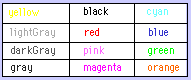### Examples :

<applet code="EqnWriter.class" archive="eqn.jar" width=600 height=330>
<param name=eqn value="diff(sin(x^2)/exp(x)^2,x)">
<param name=fgcolor value="blue">
<param name=fontName value="times">
<param name=fontSize value="16">
</applet>

<applet code="EqnWriter.class" archive="eqn.jar" width=600 height=330>
<param name=eqn value="solve(sqrt(ln(x))=ln(sqrt(x)),x)">
<param name=fgcolor value="red">
<param name=bgColor value="gray">
<param name=fontName value="geneva">
<param name=fontSize value="12">
<param name=doubleBuffer value="true">
</applet>

<applet code="EqnViewer.class" archive="eqn.jar" width=600 height=330>
<param name=eqn value="diff(sin(x)/x,x)=int(e^(2*x)*sin(x),x)+1/(x+1)">
<param name=bgColor value="0xB0B0B0">
<param name=fgcolor value="white">
<param name=sdColor value="darkGray">
<param name=fontName value="geneva">
<param name=fontSize value="12">
</applet>

### Typing Expressions

Below is a table showing you how to type expressions, and the results you will achieve.

There is also a table along side it, that shows the arithmetic keys used when building an expression.

`     `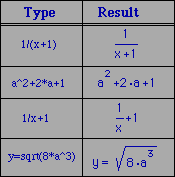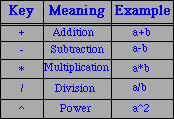### Typing Variables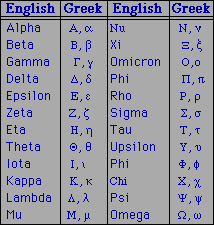For upper case Greek letters begin by typing its name using a capital letter.

You can also combine the English and Greek alphabets by using an underscore "_".

There are some Math symbols available such as infinity (inf) and imaginary (imag).

Here are some examples:

```Delta_x+Delta_y

cos(2*pi*omega+psi)

inf+imag

```

### Typing Functions

Below is a table showing some special functions. A non special function will be displayed in functional notation i.e.
funcName (param1, param2, ...), for instance: sin(pi), and perm(4,2).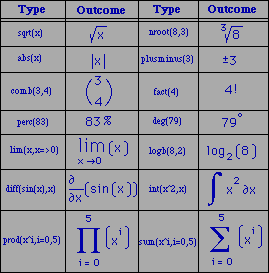### Typing Relations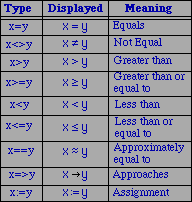The table to your right identifies all possible relations, how to type these relations and what will be seen on your web page.

### Tables and Matrices

You can also view tables and matrices. For a multiplication of two, 2x2 matrices. In your html applet;

```Type:       [sin(theta),1;-1,cos(theta)]*
transp([sin(theta),1;
-1,cos(theta)])
```

A 2x6 table is similar to the matrix, however, a single quote (') is used just after the square bracket to differentiate it from a matrix. In your html applet;

```Type:      ['x,0,pi/2,pi,(3*pi)/2,2*pi;
y,sin(0),sin(pi/2),sin(pi),sin((3*pi)/2),sin(2*pi)]
```

## Here is How EqnWriter Works

EqnWriter has the ability to dynamically build equations onto your web page. Equations can be built directly from the keyboard, while EqnWriter's syntax, like that of EqnViewer is the same used in calculators and other math software. However, the dynamic parser used by EqnWriter uses the standard precendence rules, thus you type naturally without the use of any special key combinations. EqnWriter not only has the ability of creating equations on your web page, you can also fully manipulate any equation or mathematical expression on your web page. When building equations or any type of mathematical expression, each key stroke you make is recorded in an ASCII string that can be printed to the java console by pressing enter. This ASCII string can then be copied and pasted into your html file. Upon saving your html file, you can then re-load your web page, and observe your new equations. This becomes a quick and easy way of obtaining equations and mathematical expressions on your web page.

### Clicking Modes

On your web page, you can dynamically move and select terms and expressions within your equation. You have the ability of grouping terms, and adding more expressions to your equations. To understand any of these features, the clicking modes of EqnWriter must be understood. There are essentially two clicking modes: a first click is done by moving your mouse pointer to the object, then pressing and releasing the mouse button. With a single click, you are selecting a term. A second click causes the cursor to start blinking, which then allows you to build on your equation, or delete any terms.

### Arranging Expressions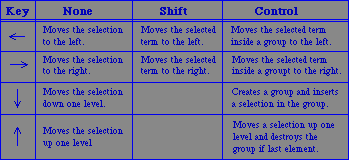The table to the right, outlines the use of the arrow keys for arranging expressions.

### Selection Modes

There are three possible selection modes that can help you manipulate any expression when using EqnWriter. First, an item can be selected by a single click on the item of interest. Second, a term can be selected by a single click of the mouse button while holding and dragging the mouse over the desired term.

EqnViewer and EqnWriter complement each other. The syntax for each are identical. That is, all the instructions provided previously for EqnViewer work also for EqnWriter. With all the new information outlined under EqnWriter, you are now fully able to write and manipulate expressions dynamically on your web page. All information about your mathematical expression is recorded in ASCII string. The nice thing about EqnWriter, you can dynamically build and manipulate any mathematical expression on your web page and view their results simultaneously.

### Last Tips

You can use ctrl-c to copy an expression to the clipboard under windows and ctrl-v to paste it.